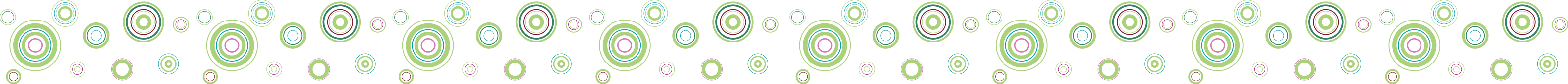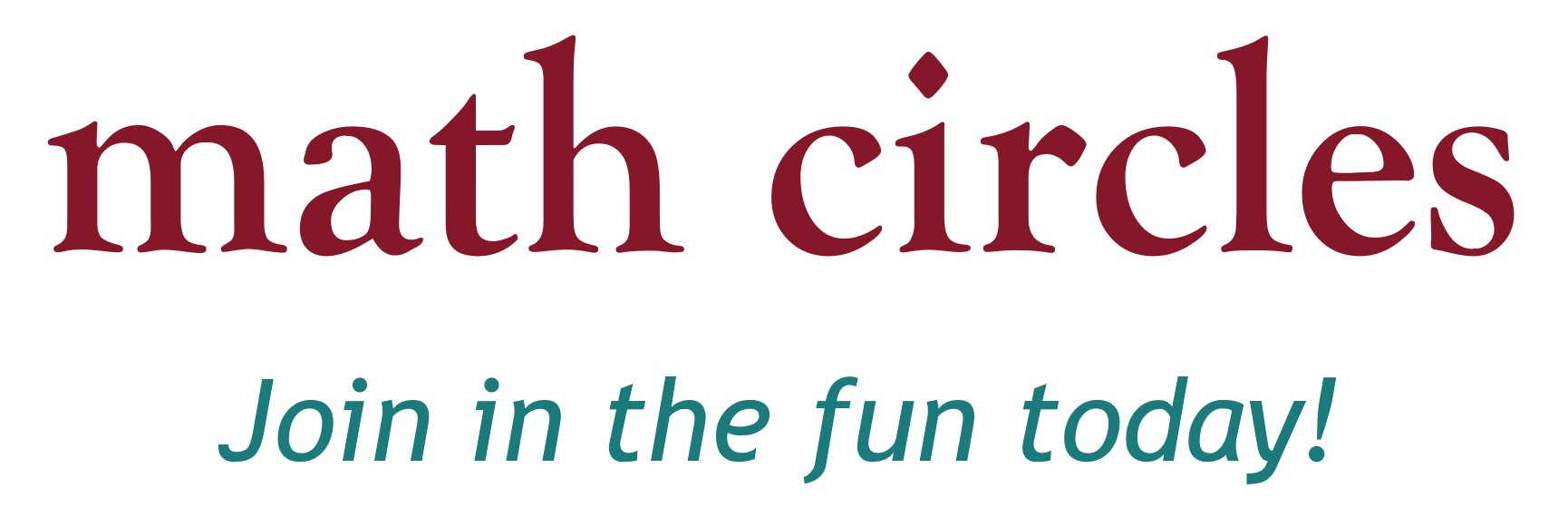Home About Us School Visits Elementary Schools Junior High Schools Senior High Schools Events at Dalhousie Virtual Classroom Visits Extra-Curricular Activities (updated) Contact Us Follow @NSMathCircles   NS Math Circles
Junior High Schools

The below is a list of presentations currently offered, appropriate for junior high school classes, in alphabetical order. If you would like us to come and give any of these presentations in your classroom for free, please use the contact form on the School Visits page.

Bothersome BrainteasersLanguage: English

Has it been a real long time since you have 'troubled' your brain in finding the right answer to a question? In this talk, you will experience four different brainteasers on shapes and patterns to tease your brain and have a good laugh too!

Outcomes:

• Hone problem solving skills.
• Explore Eulerian circuits and their mathematical properties.
• Develop intution for problems involving the infinite.

Candy GameLanguage: English

Four of you received four jars full of candies for being respectful to your teacher and your peers! But you find out that not all jars have the same number of candies, and you want to make sure that everyone in your group has the same number of candies. But there is a twist on how you can move candies around, and it's up to you to be fair to your peers in the most efficient way possible.

Outcomes:

• Study the mathematics behind the strategies to a game.
• Find leverages to come up with the most efficient method to solve a mathematical problem.
• Use logic to explain why you method is the optimal method.

Dots and Boxes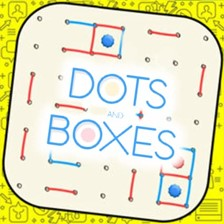Language: English

Dots and Boxes is a game invented by French mathematician Édouard Lucas, who first published a paper on it in 1895. Lucas called it la pipopipette. It is generally a two-player game, although it can be adapted to three or more players. The game is played on a grid of dots, and the goal is to form the most boxes by filling in edges between the dots. Dots and Boxes is an excellent introduction to games involving strategy. Learning this game can increase your capacity for strategic thinking, but it is also a lot of fun! We will guide you through the simple rules by going through an example, and then let you play and experiment to see what outcomes you can achieve.

Outcomes:

• Introduction to an important and deep branch of mathematics, namely Game Theory.
• Introduction to posing problems in the context of a game: i.e.: what happens if we change the rules a little bit?
• Develop strategic thinking in a fun setting.

Eulerian Circuits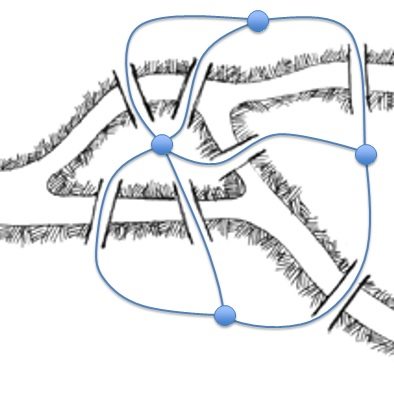Language: English

In this talk, students will be asked to find a route through the historic city of Königsberg so that they cross every bridge exactly once. This problem spawned an area of mathematics called graph theory, which makes this problem (and many others) much easier to solve! This talk serves as a gentle introduction to graph theory and its applications.

Outcomes:

• Solve problems using graphs.
• Learn definitions involving graphs.
• Look into different types of graphs.
• Try to deduce mathematical theorems.
• Understand why graph theory is so important in real life.

Fibonacci and the Golden RatioLanguage: English

In this talk students discover the relationship between the Golden Ratio and Fibonacci numbers. We also learn why the Golden Ratio is found throughout nature and listen to Fibonacci number inspired music.

Outcomes:

• What can happen when you sum numbers with a certain pattern.
• Look into a spatial math sequence and a special number, that appears in nature a lot and is now used in everyday life.
• Math and art.
• Math and music.
• Count the number of solutions to a problem.

Fractions Fun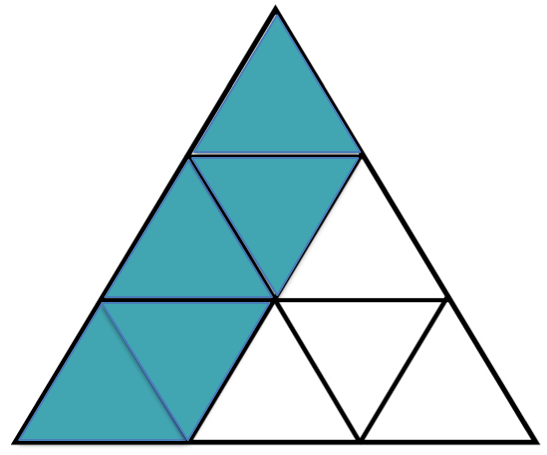Language: English

We introduce and explore the world of fractions! Using visual and real life examples as well as fraction based games we make fractions easy and fun.

Outcomes:

• Adding fractions with different denominators.
• Represent a given fraction concretely or pictorially.
• Using fraction addition, subtraction, multiplication, and division to solve problems.

This presentation is currently undergoing trials. If you are willing to host a trial-run in your classroom, please contact us.

Jury DutyLanguage: English

A crime has been committed! By exploring various areas of mathematics, such as logic, graph theory, geometry and more we will see if we can put the guilty party behind bars!

Outcomes:

• Use math to solve problems that seem to have unpredictable solutions.
• Attempt to think outside the box to solve math problems.
• Determine and explain strategies for solving problems or winning games.
• Understand that a problem might not have a solution and being capable of explaining why.
• Apply logic to solve problems.
• Learn how to eliminate possible solutions to a problem.
• Introduction the mathematical areas that are currently studied: graph theory, cryptography, logic, geometry, algebra and number theory.

This presentation is currently undergoing trials. If you are willing to host a trial-run in your classroom, please contact us.

Mathemagic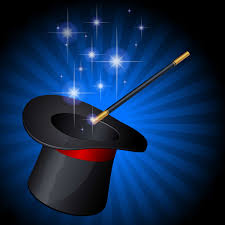Language: English

Can you always correctly guess someones birthday, magically untie yourself from ropes, or read someones mind? You should be able to! It is not magic, it's math!

Outcomes:

• Use math to solve problems that seem to have unpredictable solutions.
• Introduction to binary representation of numbers.
• Review of mental math skills and applying these to concrete problems.
• Factorization of large numbers.
• Use spatial skills to solve problems.

This presentation is currently undergoing trials. If you are willing to host a trial-run in your classroom, please contact us.

Nasty Number Tricks and Devious DivisibilityLanguage: English

G.H. Hardy (a famous 20th Century mathematiian) wrote: 'Beauty is the first test: there is no permanent place in the world for ugly mathematics'.

Let us face it-algebra can be hard and there will be point for everyone when they find using algebra is difficult. But, believe it or not, algebra can be really beautiful and is, in fact, a powerful tool! Algebra can give a great sense of achievement every time a problem is solved and can, definitely, provide effective methods to break down and solve problems. In this talk, you will feel such a satisfaction and you will see how algebra allows you to take a situation and make it more general by examining nasty number tricks and devious divisibility.

Outcomes:

• TBA.

This presentation is currently undergoing trials. If you are willing to host a trial-run in your classroom, please contact us.

Pascal's Triangle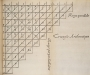Language: English

Pascal's triangle is an array of numbers arranged in a triangular shape which, upon closer inspection, contains fascinating patterns. In this presentation we explore what information Pascal's triangle contains and discover that it has connections to combinatorics ("art of counting"), number theory, geometry and even fractals. We will also see an idea that seems true, intuitively, but is surprisingly not yet proven.

Outcomes:

• Observe what happens when you sum up numbers in certain ways, and detect any patterns.
• Think about numbers geometrically and visually, and draw the connection between numbers and geometric shapes.
• Use Pascal's triangle to solve counting problems.
• Understand what it means to "show" a claim in the world of mathematics.
• Try to pose appropriate mathematical questions to explore.

Problem Solving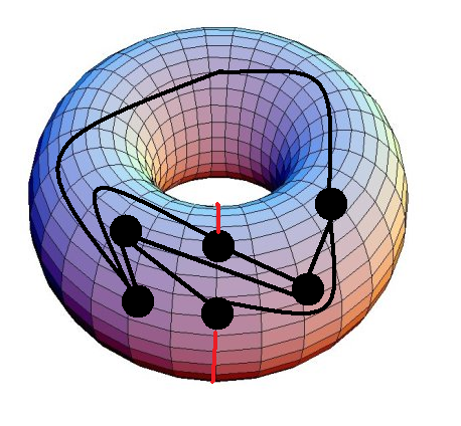Language: English

Explore the art of problem solving by investigating
tiling problems, number games and graph theory problems.

Outcomes:

• Use math to solve problems that seem to have unpredictable solutions.
• Attempt to think outside the box to solve math problems.
• Determine and explain strategies for solving problems or winning games.
• Understand that a problem might not have a solution and being capable of explaining why.
• Practice mental math skills.
• Recognize equivalent problems.
• View some interesting applications of areas and geometry.

TessellationsLanguage: English

We will explore geometry in artwork and nature through tessellations. We will look at the work of artist who used mathematics to enhance their work and even create some of our own tessellation artwork!

Outcomes:

• Counting possible solutions to a problem.
• Finding repeated patterns.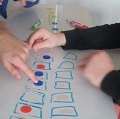Language: English

Toads & Frogs is a game that is easy to play and fun to explore. We will find the number of moves needed to solve the game, and discover more about number patterns within the game.

Outcomes:

• Study the mathematics behind the strategies to a game.
• Use formulas to express number patterns.
• Introduce polynomials.

Tower of HanoiLanguage: English

The Tower of Hanoi is a very old and very popular problem. How many moves does it take to move a stack of disks from one peg to another, following certain rules? Legend has it that priests in a temple were solving the puzzle with 64 golden disks, and when the last move is completed, the world would end!

Outcomes:

• Study a classical game.
• Find number patterns within data.
• Use formulas to express number patterns.

Contact us for ideas on how we can best serve your needs!

Contact: Reach us through our form HERE.

 Many Thanks to our Partners!Copyright www.nsmathcircles.com All Rights Reserved.